# RD Sharma Solutions for Class 8 Math Chapter 5 - Playing with Numbers (Part-1) Notes | Study RD Sharma Solutions for Class 8 Mathematics - Class 8

## Class 8: RD Sharma Solutions for Class 8 Math Chapter 5 - Playing with Numbers (Part-1) Notes | Study RD Sharma Solutions for Class 8 Mathematics - Class 8

The document RD Sharma Solutions for Class 8 Math Chapter 5 - Playing with Numbers (Part-1) Notes | Study RD Sharma Solutions for Class 8 Mathematics - Class 8 is a part of the Class 8 Course RD Sharma Solutions for Class 8 Mathematics.
All you need of Class 8 at this link: Class 8

#### Question 12: Find the remainder when 981547 is divided by 5. Do this without doing actual division.

Answer 12: If a natural number is divided by 5, it has the same remainder when its unit digit is divided by 5.Here, the unit digit of 981547 is 7. When 7 is divided by 5, remainder is 2.Therefore, remainder will be 2 when 981547 is divided by 5.

#### Question 13: Find the remainder when 51439786 is divided by 3. Do this without performing actual division.

Answer 13: Sum of the digits of the number 51439786 = 5 + 1 + 4 + 3 + 9 + 7 + 8 + 6 = 43

The remainder of 51439786, when divided by 3, is the same as the remainder when the sum of the digits is divided 3.

When 43 is divided by 3, remainder is 1.

Therefore, when 51439786 is divided by 3, remainder will be 1.

#### Question 18: Solve each of the following Cryptarithms: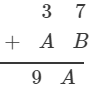#### Question 19: Solve each of the following Cryptarithm: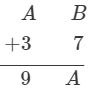#### Question 20: Solve each of the following Cryptarithm: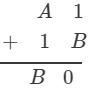#### Question 21: Solve each of the following Cryptarithm: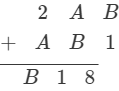#### Question 22: Solve each of the following Cryptarithm: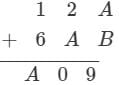#### Question 23: Solve each of the following Cryptarithm: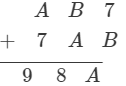#### Question 24: Solve each of the following Cryptarithm: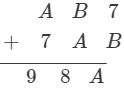#### Answer 24: 0 is the only unit digit number, which gives the same 0 at the unit digit when multiplied by 4. So, the possible value of B is 0.Similarly, for A also, 0 is the only possible digit.But then A, B and C will all be 0.And if A, B and C become 0, these numbers cannot be of two−digit or three−digit.Therefore, both will become a one−digit number.Thus, there is no solution possible.

The document RD Sharma Solutions for Class 8 Math Chapter 5 - Playing with Numbers (Part-1) Notes | Study RD Sharma Solutions for Class 8 Mathematics - Class 8 is a part of the Class 8 Course RD Sharma Solutions for Class 8 Mathematics.
All you need of Class 8 at this link: Class 8Use Code STAYHOME200 and get INR 200 additional OFF

## RD Sharma Solutions for Class 8 Mathematics

88 docs

Track your progress, build streaks, highlight & save important lessons and more!

,

,

,

,

,

,

,

,

,

,

,

,

,

,

,

,

,

,

,

,

,

;